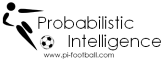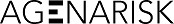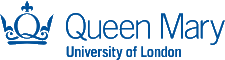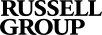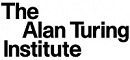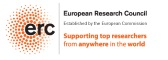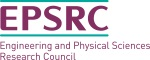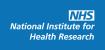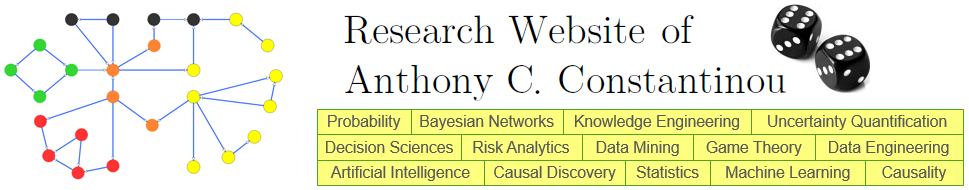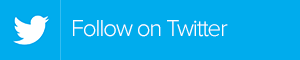: Home : Interactive Calculators : Risk of Ruin

 Risk of Ruin Calculator It is always wise to know the risks involved with your initial bankroll when gambling or trading. The risk of ruin (also known as the probability of ruin) refers to the probability of turning a specified amount of money into nothing, and it is very popular amongst professionals in Blackjack, Poker and financial traders; but also perfectly suitable for many other forms of gambling. It appears that the Risk of Ruin was first presented in  by the British statistician David R. Cox and Hilton D. Miller. The probability is calculated given your current bankroll, the profit/win rate per trial/trade (or per minute/hour), and the standard deviation of the winnings. This is expressed as: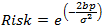where e is the Euler's Number ~2.7182, b  is the amount of money (bankroll) whose risk of losing you are evaluating, p is your profit ratio per trial and σ is the standard deviation of winnings. Bankroll (e.g. in €) Profit rate per trial (e.g. input of 0.10 for 10%) Standard Deviation of profit rate . With a bankroll of N/A , a profit rate of N/A  per trial, and a σ of  N/A ; your risk of ruin is N/A %. Note that this calculator assumes that your profit rate and standard deviation will not change over time; implying that the accuracy of the result is dependent on the confidence of your inputs (e.g. you are much more confident when your profit rate and standard deviation are determined by thousands of trials rather that hundreds). Further, the resulting probability refers to the specified risk for infinite amount of trials, which is considered as a disadvantage by many since it is impossible for a gambler to bet/trade that long. Despite the limitations, the generated result is a reasonable estimate for controlling your risk and certainly superior to "advices". Alternatively, If you are looking for the size of bankroll required for n probability of ruin, this is expressed as:where e is the Euler's Number ~2.7182, r  is the risk of ruin, p is your profit ratio per trial, σ is the standard deviation of winnings and ln() is the natural logarithm Probability of risk of ruin (e.g. input of 0.10 for 10%) Profit rate per trial (e.g. input of 0.10 for 10%) Standard Deviation of profit rate . For a probability of ruin of N/A , with a profit rate of N/A  per trial, and with a σ of  N/A ; your required bankroll is N/A €. _______________________________________ References  Cox, D. R., & Miller, H. D. (1965). The theory of stochastic processes. Methuen, London. ___________________________________________________________________________________________ published online, 19/02/2012